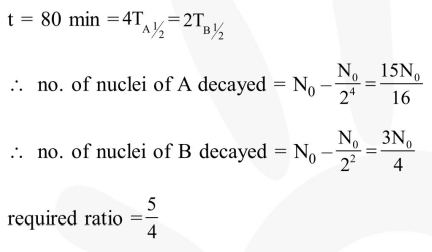# Half-lives of two radioactive elements`
Question:

Half-lives of two radioactive elements $\mathrm{A}$ and $\mathrm{B}$ are 20 minutes and 40 minutes, respectively. Initially, the samples have equal number of nuclei. After 80 minutes, the ratio of decayed numbers of $A$ and $B$ nuclei will be :-

1. $5: 4$

2. $1: 16$

3. $4: 1$

4. $1: 4$

Correct Option: 1

Solution: